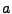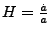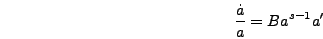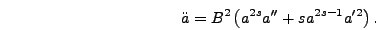Next: Spectra Up: Output Previous: Field Means and Variances

# The Scale Factor and Its Derivatives

The function scale() outputs the scale factor, the Hubble constant, and the second derivative. These quantities are all output in physical units. Using the rescalings in equation (6.2) these can be written in terms of program variables as(5.2)(5.3)

The file scale_ext contains one column for the time and three for these three quantities, in the order just given. Note that even if sscale is set to one this function is only called if self-consistent expansion is used. If there is no expansion then there is no scale factor evolution and for power-law expansion this evolution is simply given by a fixed equation.